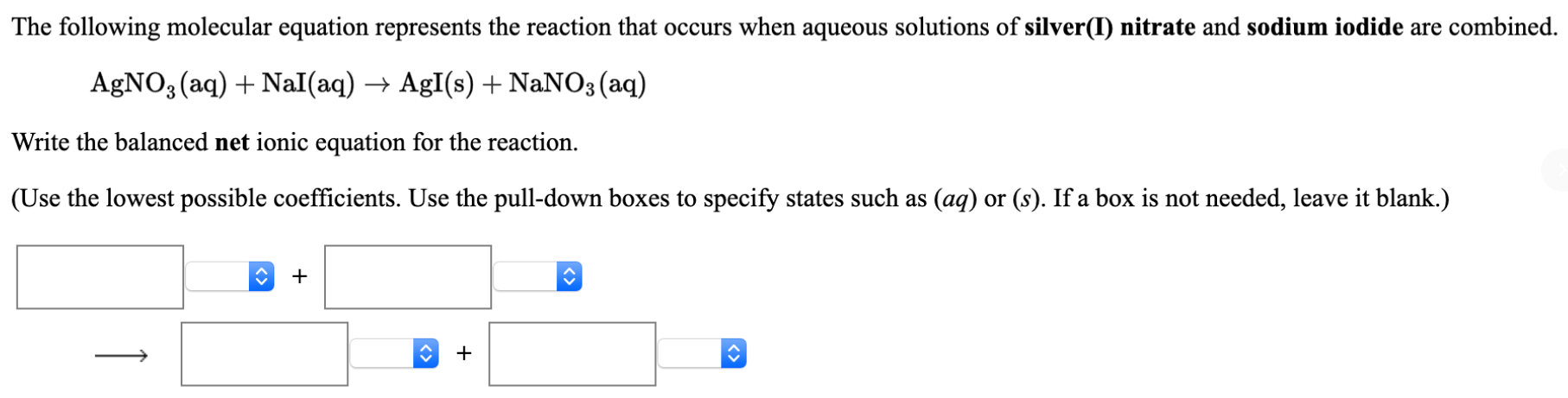# The following molecular equation represents the reaction that occurs when aqueous solutions of silver (I) nitrate and sodium iodide are combined. AgNO3(aq) + NaI(aq) → AgI(s) + NaNO3 (aq) Write the balanced net ionic equation for the reaction. (Use the lowest possible coefficients. Use the pull-down boxes to specify states such as (aq) or (s) . If a box is not needed, leave it blank.)